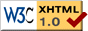# Draft Proposal for UTF-E-16 Specification

Authors: Tom Bishop (tbishop@wenlin.com) and Richard Cook (rscook@wenlin.com).

Updated October, 2009

Please Note: This is not a finalized specification. It is still at the "draft proposal" stage and may change.

## 1. Introduction

The name of this UTF (UCS Transformation Format) is "UTF-E-16". It extends UTF-16 to support code points up to U+7FFFFFFFFFFFFFFF.

UTF-E-16 is one of the encodings defined as part of UCS-E, which also includes similar extensions for UTF-8 and UTF-32. For general information about UCS-E, please see the UCS-E Specification.

## 2. Properties

UTF-E-16 preserves and extends useful properties of UTF-16 and UTF-G-16. For code points less than or equal to U+10FFFF, it is identical to UTF-16. For code points less than or equal to U+7FFFFFFF, it is identical to UTF-G-16.

Codes for code points greater than or equal to U+80000000 have four to eight units, all in the range DC04..DFFF. In other respects, UTF-E-16 shares the same properties listed in the UTF-G-16 specification.

## 3. Details

(Note: some readers may prefer to skip down to view the examples first, then return here to read the details.)

### 3.1 Terms and Abbreviations

• Code point: Any nonnegative integer used for character encoding.
• USV: A UCS-E scalar value, that is, any code point in the range U+0000..U+7FFFFFFFFFFFFFFF except U+D800..U+DFFF.
• Udigit: A USV hexadecimal digit used in the conventional "U+" notation.
• NUD: Number of udigits in a particular USV, not counting leading zeros. For example, U+123456789 has NUD = 9, and U+0050 has NUD = 2.
• Unit: A sixteen-bit code unit that forms part or all of a UTF-E-16 code.
• Code: A whole sequence of UTF-E-16 units corresponding to a single code point.

### 3.2 Code points less than or equal to U+10FFFF

For code points less than or equal to U+10FFFF, UTF-E-16 is identical to UTF-16. Codes are either one unit (up to U+FFFF) or two units (above U+FFFF, using surrogate pairs).

### 3.3 Code points in the range U+110000..U+7FFFFFFFFFFFFFFF

3.3.1 For code points in the range U+110000..U+7FFFFFFFFFFFFFFF (fifteen F's), a code consists of between three and eight units. The leading unit is in the range DC04..DDF0 and conforms to the bit pattern 1101110xxxxxxxxx (binary), where the nine x bits indicate the number of units as follows:

```        Bit pattern of low nine       Number
bits in leading unit       of units
0yyyyyyyy .............. 3
10yyyyyyy .............. 4
110yyyyyy .............. 5
1110yyyyy .............. 6
11110yyyy .............. 7
111110yyy .............. 8
```

3.3.2 The bits marked y in the table are available for storage of the USV.

3.3.3 Trailing units are in the range DE00..DFFF, with bit pattern 1101111xxxxxxxxx (binary), where the nine x bits are available for storage of the USV.

3.3.4 The USV is stored in the available bits. A code always uses the minimum number of units necessary to store the USV. Leading zero bits on the first udigit are omitted if this omission results in a shorter code. (For example, the first udigit of U+1FFFFFF is 1, which in binary is 0001, with three leading zero bits which are NOT all stored in the code.) If more bits are available than are needed to store the USV, then the USV is effectively padded on the left with zero bits to fill the leftover space.

### 3.4 Analysis

2 unitsU+10000..U+10FFFFD800..DBFFDC00..DFFF
3 unitsU+110000..U+3FFFFFFDC04..DCFFDE00..DFFF
4 unitsU+4000000..U+3FFFFFFFFDD00..DD7F
5 unitsU+400000000..U+3FFFFFFFFFFDD80..DDBF
6 unitsU+40000000000..U+3FFFFFFFFFFFFDDC0..DDDF
7 unitsU+4000000000000..U+3FFFFFFFFFFFFFFDDE0..DDEF
8 unitsU+400000000000000..U+7FFFFFFFFFFFFFFFDDF0..DDF0

3.4.1 The table above illustrates the forms of 2-to-8-unit codes (as already defined in sections 3.2 and 3.3).

LengthD800..DBFFDC00..DDFFDE00..DFFF

3.4.2 The second table rearranges some of the same information. It shows that leading units can be distinguished from trailing units based on their values, except for some surrogates in the range DC00..DDFF that can function either as trailing units in 2-unit codes, or as leading units in longer codes. Units DC00..DDFF can be recognized as leading or trailing by the same two rules that apply to UTF-G-16:

• A unit DC00..DDFF is trailing if (and only if) it is immediately preceded by a unit D800..DBFF.
• A unit DC00..DDFF is leading if (and only if) it is immediately followed by a unit DE00..DFFF.

Either rule can be applied, whichever is most convenient; the results are the same for well-formed UTF-E-16 text. (See the function `isLeadingUTFG16Unit()` in ConvertUTFG.c for an implementation of these rules.)

3.4.3 Only part of the range DC00..DDFF, namely DC04..DDF0, is used for leading units in UTF-E-16. (The two rules in 3.4.2 are formulated to cover further extensions, such as UTF-∞-16, which may use DDF1..DDFF as leading units.)

## 4. Examples

``` U+0041 = 0041 (one unit; the code for the letter 'A')

U+10FFFF = DBFF DFFF (two units; the last UTF-16 code, composed of two surrogates)

U+110000 = DC04 DE80 DE00 (three units; the first code that is not UTF-16)

U+3FFFFFF = DCFF DFFF DFFF (the last three-unit code)

U+4000000 = DD00 DF00 DE00 DE00 (the first four-unit code)

U+7FFFFFFF = DD0F DFFF DFFF DFFF (four units; the last code point in UCS-G)

U+80000000 = DD10 DE00 DE00 DE00 (the first code point beyond UCS-G)

U+3FFFFFFFF = DD7F DFFF DFFF DFFF (the last four-unit code; NUD = 9;
3FFFFFFFF hex = 17,179,869,183 decimal)

U+123456789ABCD = DDC9 DE34 DEAC DFE2 DED5 DFCD (six units; NUD = thirteen)

U+7FFFFFFFFFFFFFFF = DDF0 DFFF DFFF DFFF DFFF DFFF DFFF DFFF
(eight units; NUD = sixteen; the last code point in UCS-E)
```

To the UCS-E Specification